## Investigation of Copper(II) amino acid complexes

A metabolism for copper ions is widespread among living systems. To help in the understanding of the binding of the ion in biopolymers and physiological fluids, the prototype compounds of cupric ions with amino acids have been extensively studied.
Bis (glycinato)copper(II).hydrate was first isolated in 1841 and in 1890 a second form was discovered. It was later suggested that the two modifications; one pale blue needles and the other bluish violet glistening plates were geometric (cis/trans) isomers.
These compounds represent some of the first geometric isomers discovered and recently the novel solid state thermal isomerisation of the cis- to the trans- form was reported.
In this experiment, the formation of the copper(II) glycine complexes will be studied as a function of pH and from the titration data the overall stability constant b2 will be calculated. In addition, IR spectroscopy will be used in conjunction with a simple group theory approach to distinguish between the cis- and trans- isomers of Cu(gly)2.H2O.

### Part A-Stability Constant Calculations

The formation of metal complexes is often highly dependent on the pH of the solution. This is because there is a competition for the ligand between the metal ion and the proton as they both bind to the same atoms of the ligand. For glycine at low pH, the metal ion has to displace a proton from the amine N in order to form a stable complex.
```      Cu2+(aq)    +    2H3N+CH2COO-   ⇔   Cu(NH2CH2COO)2    +   2H+(aq)
```

The number of protons displaced through chelate formation can be determined from a pH titration of a mixture of ligand and metal ion; in this instance a 2:1 mixture of glycine and Cu2+(aq) is used. It is also necessary to do a pH titration of glycine alone, so that the dissociation constants of the ligand can be obtained.

For the ligand:

```        H3N+CH2COOH   ⇔   H3N+CH2COO- (gly-)  +   H+(aq)     Ka1
H3N+CH2COO-   ⇔   H2NCH2COO-          +   H+(aq)     Ka2
```
The stepwise stability constants K1 and K2 for the reaction of the ligand with Cu2+ are given by the expressions:
```  Cu2+        + gly-  ⇔ Cu(gly)+  K1 (β1)
Cu(gly)+    + gly-  ⇔ Cu(gly)2  K2
```
and the overall stability constant β2 = K1 * K2

From the titration curve for glycine, the pH at 0.5 the equivalence point gives pKa1 while at 1.5 gives pKa2.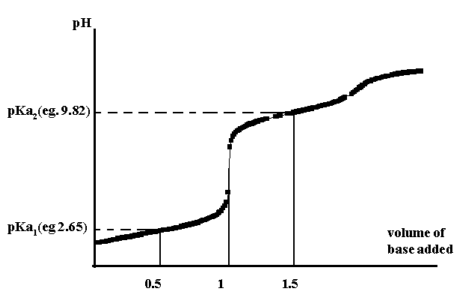Figure 1.

To calculate the stability constants (K1 and K2) use is made of the formation function, n, which is defined as the ratio of total ligand bound to the total metal present:

```
n = { [Cu(gly)+] + 2[Cu(gly)2] } / { [Cu2+] + [Cu(gly)+] + [Cu(gly)2] }
```
substituting from equations (1) and (2) gives
```  n  =  { β1(gly-) + 2β2 [gly-]2 } / {1 + β1(gly-) + β2 [gly-]2}
```
From an experimental standpoint, this needs to be expressed in terms of total copper(II) ions, total glycine and pH for the calculation to be able to be carried out. When this is done, the expression becomes:
```    [Hgly] = [Hgly]total  - [H+] -  [Na+] + [OH-] / (1+[H+]/Ka1)

and  n  = {[Hgly]total - [Hgly]}.{1+Ka2/[H+]+[H+]/Ka1} /[Cu2+]total

also    [gly-] = Ka2.[Hgly]/[H+]
```
if n versus p(gly-) is plotted, then an approximate value of the stepwise formation constants, K1 and K2, are obtained at =0.5 and =1.5. A more accurate measure of β2 is obtained at =1.0.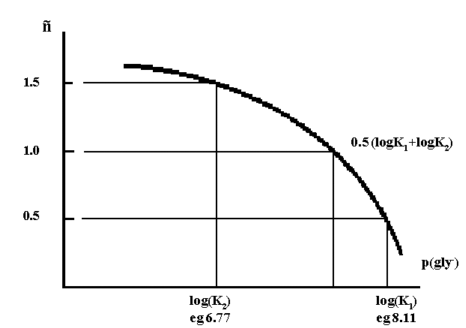Figure 2.

A more precise method uses a simple linear least squares analysis of the titration results and makes use of the equation:

```  n = {β1[gly-] + 2 β2 [gly-]2} / { 1+ β1[gly-] + β2[gly-]2 }
```
Rearrangement of which gives:
```  n/((1-n)[gly-])  = β1 +  β2 * (2-n) * [gly-]/(1-n)

Thus a plot of  n/((1-n)[gly-]) versus (2-n)[gly-]/(1-n)
```
should yield a straight line. Most programmable calculators can do a least squares fit, else it can be done easily with a spreadsheet application such as Excel.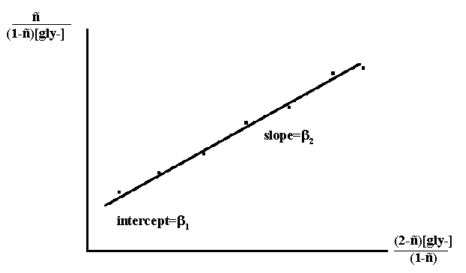Figure 3.

## Procedure.

Prepare the following stock solutions:
a) 500 cm3 of 0.1M NaOH. This should be standardised with potassium hydrogen phthalate.
b) 500 cm3 of 0.1M HNO3. This should be standardised with the 0.1M NaOH above.
c) 10 cm3 of 0.1M Cu(NO3)2 solution from 0.25g Cu(NO3)2.2.5H2O.
d) 250 cm3 of 0.05M KNO3.

### Titration of Glycine

Weigh (to the nearest 0.1mg) a 0.1g sample of glycine into a 250 cm3 beaker. Pipette 100 cm3 of 0.05 KNO3 into the beaker, mix and record the pH. Pipette 10 cm3 of standardised 0.1M HNO3 into the beaker and record the new pH of the solution. Titrate the solution with the standardised 0.1M NaOH, making sure to stir continuously after each addition and to plot the titration curve AS YOU PROCEED. Continue the titration until the number of moles of base added is equal to the number of moles of HNO3 plus the number of moles of glycine present.

### Titration of the Copper-Glycine system

Weigh (to the nearest 0.1mg) a 0.1g sample of glycine into a 250 cm3 beaker. Pipette 100 cm3 of 0.05 KNO3 into the beaker, mix and record the pH. Add by pipette, 2 cm3 of 0.1M copper(II) solution and record the pH again. Titrate, using ~ 0.2 cm3 increments of standard 0.1M NaOH, with continuous stirring. Note: the calculations give the best results when the first five or six data points from the titration curve are used.

### Preparations.

#### cis-Cu(gly)2.H2O

Copper(II) acetate monohydrate (0.01 mole, 2.0 g) is dissolved in 25 cm3 of hot water. 25 cm3 of hot ethanol is added and the solution kept hot. Glycine(0.02 mole, 1.5 g) is dissolved in 25 cm3 of hot water. The solutions are mixed while hot (~70C) and the solution then cooled on ice. A needle-like precipitate is obtained. Record the yield. Preserve the filtrate.

#### trans-Cu(gly)2.H2O

Two methods have been used for the preparation of the trans- isomer, but the first gives the cleaner product.
a) 10 cm3 of the filtrate above is placed in a small flask with ~ 1.5 g of the cis- isomer and 1 g of glycine. The suspension is heated under reflux for one hour. The hot mixture is then filtered to give the trans-Cu(gly)2.H2O. Note that if the mixture all dissolves during the reflux, then the cis-product will reprecipitate not the trans-.
b) An alternative method, is to place a small quantity of the cis-isomer in an oven at 170¦C for an hour. On cooling in air, this leads to the solid state conversion to the trans-isomer.

## Part B IR spectra of cis- and trans- Cu(gly)2. H2O

It should be possible to distinguish between the two isomers by considering the immediate environment around the central metal and assuming that their vibrations are independent of the motions involving the rest of the atoms of the ligands.
IR spectra (KBr pellet 4400-400 cm-1) in JCAMP-DX format
cis-isomer
trans-isomer
That is, by considering only the Cu and the N and O atoms, the cis-isomer will have C2v symmetry, whilst the trans-isomer D2h symmetry.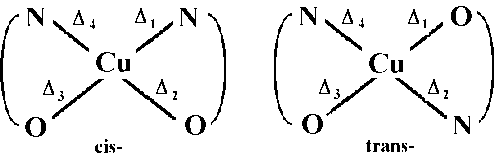Figure 4.

The reducible representations for C2v and D2h are: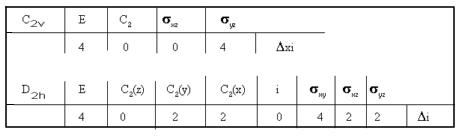Figure 5.

and using the reduction formula, then for:

```       C2v     →   2A1   +    2B2            all bands should be IR active,
while for
D2h     →   2A1g  +     B2u    +   B3u  only the B2u and B3u bands

should be IR active, the 2A1g symmetric bands being Raman active.
```
It is expected therefore that for the metal-ligand stretching vibrations, 4 bands should be observed in the IR spectrum of the cis-isomer, but only 2 bands for the trans-isomer. Since N is the lighter atom it is also expected that the M-N modes will be at higher energy than the M-O modes.

### Interpretation.

The metal-ligand stretching modes occur below 500 cm-1. From the spectra in the Appendix tabulate all peaks in that region. (Note: the band at ~380 cm-1 is common to both spectra and has been described as a skeletal band and will not be used in the interpretation).
For the cis-isomer, assign v asymmetric and v symmetric bands for M-N and M-O. For the trans-isomer, assign v asymmetric for M-N and M-O.
From the spectra recorded on your samples (4000-600 cm-1), tabulate the major peaks and assign as many bands as possible. Can you make use of another region in these spectra to distinguish between the two isomers?

### Questions.

1) The use of Cu(II) salts, including those of simple amino-acids, has been described for the treatment of rheumatoid arthritis. How is the copper thought to act? Give two references outlining this work.
2) A number of copper containing enzymes are known. Give the names of two of these and show the coordination environment around the copper.
3) It has been claimed that to a good approximation
```      log K1 = pKa2-pH-log([L] - 1/2 [Cu])
```
where [L]=total ligand concentration in all forms and
[Cu]=total copper concentration in all forms
pKa1 is the value for glycine and pH is the pH of the copper glycine mixture when one quarter of the glycine has been titrated.

Also that

```      log K2 = pKa2-pH-log([L] - 3/4 [Cu])
```
where the pH is now taken when three quarters of the glycine is titrated.
Use your titration results to show whether this approximation gives reasonable results.

## References

```  J.J. Farrell, J. Chem. Educ., 54, 1977, 445.

P. O'Brien, J. Chem. Educ., 59, 1982, 1052.

```